# Sobolev Duals for Random Frames and ΣΔ Quantization of Compressed Sensing Measurements

## Abstract

Quantization of compressed sensing measurements is typically justified by the robust recovery results of Candès, Romberg and Tao, and of Donoho. These results guarantee that if a uniform quantizer of step size δ is used to quantize m measurements y=Φx of a k-sparse signal x∈ℝN, where Φ satisfies the restricted isometry property, then the approximate recovery x # via 1-minimization is within O(δ) of x. The simplest and commonly assumed approach is to quantize each measurement independently. In this paper, we show that if instead an rth-order ΣΔ (Sigma–Delta) quantization scheme with the same output alphabet is used to quantize y, then there is an alternative recovery method via Sobolev dual frames which guarantees a reduced approximation error that is of the order δ(k/m)(r−1/2)α for any 0<α<1, if m r,α k(logN)1/(1−α). The result holds with high probability on the initial draw of the measurement matrix Φ from the Gaussian distribution, and uniformly for all k-sparse signals x whose magnitudes are suitably bounded away from zero on their support.

This is a preview of subscription content, access via your institution.

1. 1.

The quantization error is often modeled as white noise in signal processing, hence the terminology. However our treatment of quantization error in this paper is entirely deterministic.

2. 2.

The optimality remark does not apply to the case of infinite quantization alphabet $$\mathcal {A}= \delta \mathbb {Z}$$ because depending on the coding algorithm, the (effective) bit-rate can still be unbounded. Indeed, arbitrarily small reconstruction error can be achieved with a (sufficiently large) fixed value of λ and a fixed value of δ by increasing the order r of the ΣΔ modulator. This would not work with a finite alphabet because the modulator will eventually become unstable. In practice, almost all schemes need to use some form of finite quantization alphabet.

3. 3.

As mentioned earlier, we do not normalize the measurement matrix Φ in the quantization setting.

4. 4.

Balls with radii ρ/2 and centers at a ρ-distinguishable set of points on the unit sphere are mutually disjoint and are all contained in the ball of radius 1+ρ/2 centered at the origin. Hence there can be at most (1+ρ/2)k/(ρ/2)k of them.

## References

1. 1.

R. Baraniuk, M. Davenport, R. DeVore, M. Wakin, A simple proof of the restricted isometry property for random matrices, Constr. Approx. 28(3), 253–263 (2008).

2. 2.

J.J. Benedetto, A.M. Powell, Ö. Yılmaz, Second order sigma–delta (ΣΔ) quantization of finite frame expansions, Appl. Comput. Harmon. Anal. 20, 126–148 (2006).

3. 3.

J.J. Benedetto, A.M. Powell, Ö. Yılmaz, Sigma–delta (ΣΔ) quantization and finite frames, IEEE Trans. Inf. Theory 52(5), 1990–2005 (2006).

4. 4.

R. Bhatia, Matrix Analysis. Graduate Texts in Mathematics, vol. 169 (Springer, New York, 1997).

5. 5.

J. Blum, M. Lammers, A.M. Powell, Ö. Yılmaz, Sobolev duals in frame theory and sigma–delta quantization, J. Fourier Anal. Appl. 16(3), 365–381 (2010).

6. 6.

B.G. Bodmann, V.I. Paulsen, S.A. Abdulbaki, Smooth frame-path termination for higher order sigma–delta quantization, J. Fourier Anal. Appl. 13(3), 285–307 (2007).

7. 7.

P. Boufounos, R.G. Baraniuk, 1-bit compressive sensing. In: 42nd Annual Conference on Information Sciences and Systems (CISS), pp. 19–21.

8. 8.

P. Boufounos, R.G. Baraniuk, Sigma delta quantization for compressive sensing, in Wavelets XII, ed. by D. Van de Ville, V.K. Goyal, M. Papadakis. Proceedings of SPIE, vol. 6701 (SPIE, Bellingham, 2007). Article CID 670104.

9. 9.

E.J. Candès, Compressive sampling, in International Congress of Mathematicians, vol. III (Eur. Math. Soc., Zürich, 2006), pp. 1433–1452.

10. 10.

E.J. Candès, J. Romberg, T. Tao, Signal recovery from incomplete and inaccurate measurements, Commun. Pure Appl. Math. 59(8), 1207–1223 (2005).

11. 11.

E.J. Candès, J. Romberg, T. Tao, Robust uncertainty principles: exact signal reconstruction from highly incomplete frequency information, IEEE Trans. Inf. Theory 52(2), 489–509 (2006).

12. 12.

A. Cohen, W. Dahmen, R. DeVore, Compressed sensing and best k-term approximation, J. Am. Math. Soc. 22(1), 211–231 (2009).

13. 13.

W. Dai, O. Milenkovic, Information theoretical and algorithmic approaches to quantized compressive sensing, IEEE Trans. Commun. 59(7), 1857–1866 (2011).

14. 14.

I. Daubechies, R. DeVore, Approximating a bandlimited function using very coarsely quantized data: a family of stable sigma–delta modulators of arbitrary order, Ann. Math. 158(2), 679–710 (2003).

15. 15.

D.L. Donoho, Compressed sensing, IEEE Trans. Inf. Theory 52(4), 1289–1306 (2006).

16. 16.

D.L. Donoho, For most large underdetermined systems of equations, the minimal l1-norm near-solution approximates the sparsest near-solution, Commun. Pure Appl. Math. 59(7), 907–934 (2006).

17. 17.

A.K. Fletcher, S. Rangan, V.K. Goyal, Necessary and sufficient conditions for sparsity pattern recovery, IEEE Trans. Inf. Theory 55(2009), 5758–5772 (2009).

18. 18.

V.K. Goyal, M. Vetterli, N.T. Thao, Quantized overcomplete expansions in ℝN: analysis, synthesis, and algorithms, IEEE Trans. Inf. Theory 44(1), 16–31 (1998).

19. 19.

V.K. Goyal, A.K. Fletcher, S. Rangan, Compressive sampling and lossy compression, IEEE Signal Process. Mag. 25(2), 48–56 (2008).

20. 20.

C.S. Güntürk, One-bit sigma-delta quantization with exponential accuracy, Commun. Pure Appl. Math. 56(11), 1608–1630 (2003).

21. 21.

L. Jacques, D.K. Hammond, J.M. Fadili, Dequantizing compressed sensing: when oversampling and non-Gaussian constraints combine, IEEE Trans. Inf. Theory 57(1), 559–571 (2011).

22. 22.

M.C. Lammers, A.M. Powell, Ö. Yılmaz, On quantization of finite frame expansions: sigma–delta schemes of arbitrary order, Proc. SPIE 6701, 670108 (2007).

23. 23.

M. Lammers, A.M. Powell, Ö. Yılmaz, Alternative dual frames for digital-to-analog conversion in sigma–delta quantization, Adv. Comput. Math. 32(1), 73–102 (2010).

24. 24.

J.N. Laska, P.T. Boufounos, M.A. Davenport, R.G. Baraniuk, Democracy in action: quantization, saturation, and compressive sensing, Appl. Comput. Harmon. Anal. 31(3), 429–443 (2011).

25. 25.

G.G. Lorentz, M. von Golitschek, Y. Makovoz, Constructive Approximation. Grundlehren der Mathematischen Wissenschaften [Fundamental Principles of Mathematical Sciences], vol. 304 (Springer, Berlin, 1996). Advanced problems.

26. 26.

D. Needell, J. Tropp, CoSaMP: iterative signal recovery from incomplete and inaccurate samples, Appl. Comput. Harmon. Anal. 26(3), 301–321 (2009).

27. 27.

D. Needell, R. Vershynin, Uniform uncertainty principle and signal recovery via regularized orthogonal matching pursuit, Found. Comput. Math. 9, 317–334 (2009). doi:10.1007/s10208-008-9031-3.

28. 28.

S.R. Norsworthy, R. Schreier, G.C. Temes (eds.), Delta–Sigma Data Converters (IEEE Press, New York, 1997).

29. 29.

R.J. Pai, Nonadaptive lossy encoding of sparse signals. PhD thesis, Massachusetts Institute of Technology (2006).

30. 30.

S.V. Parter, On the distribution of the singular values of Toeplitz matrices, Linear Algebra Appl. 80, 115–130 (1986).

31. 31.

M. Rudelson, R. Vershynin, Smallest singular value of a random rectangular matrix, Commun. Pure Appl. Math. 62(12), 1595–1739 (2009).

32. 32.

G. Strang, The discrete cosine transform, SIAM Rev., 135–147 (1999).

33. 33.

J.Z. Sun, V.K. Goyal, Optimal quantization of random measurements in compressed sensing, in IEEE International Symposium on Information Theory. ISIT 2009, (2009), pp. 6–10.

34. 34.

R. Tibshirani, Regression shrinkage and selection via the lasso, J. R. Stat. Soc., Ser. B, Stat. Methodol. 58(1), 267–288 (1996).

35. 35.

J. von Neumann, Distribution of the ratio of the mean square successive difference to the variance, Ann. Math. Stat. 12(4), 367–395 (1941).

36. 36.

M.J. Wainwright, Information-theoretic limits on sparsity recovery in the high-dimensional and noisy setting, IEEE Trans. Inf. Theory 55(12), 5728–5741 (2009).

37. 37.

M.J. Wainwright, Sharp thresholds for high-dimensional and noisy sparsity recovery using l1-constrained quadratic programming (Lasso), IEEE Trans. Inf. Theory 55(5), 2183–2202 (2009).

38. 38.

A. Zymnis, S. Boyd, E. Candes, Compressed sensing with quantized measurements, IEEE Signal Process. Lett. 17(2), 149 (2010).

## Acknowledgements

The authors would like to thank Ronald DeVore and Vivek Goyal for valuable discussions. We thank the American Institute of Mathematics and Banff International Research Station for hosting two meetings where this work was initiated. This work was supported in part by: National Science Foundation Grant CCF-0515187 (Güntürk), Alfred P. Sloan Research Fellowship (Güntürk), National Science Foundation Grant DMS-0811086 (Powell), the Academia Sinica Institute of Mathematics in Taipei, Taiwan (Powell), a Pacific Century Graduate Scholarship from the Province of British Columbia through the Ministry of Advanced Education (Saab), a UGF award from the UBC (Saab), an NSERC (Canada) Discovery Grant (Yılmaz), and an NSERC Discovery Accelerator Award (Yılmaz).

## Author information

Authors

### Corresponding author

Correspondence to Ö. Yılmaz.

Communicated by Emmanuel Candès.

## Appendix: Singular Values of D −r

### Appendix: Singular Values of D−r

It will be more convenient to work with the singular values of D r. Note that, because of our convention of descending ordering of singular values, we have

$$\sigma_j \bigl(D^{-r}\bigr) = \frac{1}{\sigma_{m+1-j}(D^r)},\quad j=1,\ldots,m.$$
(72)

For r=1, an explicit formula is available [32, 35]. Indeed, we have

$$\sigma_j(D) = 2\cos \biggl(\frac{\pi j}{2m+1} \biggr), \quad j=1,\ldots,m,$$
(73)

which implies

$$\sigma_j \bigl(D^{-1}\bigr) = \frac{1}{2\sin (\frac{\pi (j-1/2)}{2(m+1/2)} )}, \quad j=1,\ldots,m.$$
(74)

The first observation is that σ j (D r) and (σ j (D))r are different, because D and D do not commute. However, this becomes insignificant as m→∞. In fact, the asymptotic distribution of $$(\sigma_{j}(D^{r}))_{j=1}^{m}$$ as m→∞ is rather easy to find using standard results in the theory of Toeplitz matrices: D is a banded Toeplitz matrix whose symbol is f(θ)=1−e , hence the symbol of D r is (1−e )r. It then follows by Parter’s extension of Szegö’s theorem  that for any continuous function ψ, we have

$$\lim_{m \to\infty} \frac{1}{m} \sum_{j=1}^m \psi\bigl( \sigma_j\bigl(D^r\bigr) \bigr) = \frac{1}{2\pi} \int_{-\pi}^\pi\psi\bigl(\bigl|f( \theta)\bigr|^r\bigr) \,\mathrm{d}\theta.$$
(75)

We have |f(θ)|=2sin|θ|/2 for |θ|≤π, hence the distribution of $$(\sigma_{j}(D^{r}))_{j=1}^{m}$$ is asymptotically the same as that of 2rsinr(πj/2m), and consequently, we can think of σ j (D r) roughly as (2rsinr(πj/2m))−1. Moreover, we know that $$\|D^{r}\|_{\mathrm{op}} \leq\|D\|^{r}_{\mathrm{op}} \leq2^{r}$$, hence σ min(D r)≥2r.

When combined with known results on the rate of convergence to the limiting distribution in Szegö’s theorem, the above asymptotics could be turned into an estimate of the kind given in Proposition 3.2, perhaps with some loss of precision. Here we shall provide a more direct approach which is not asymptotic, and works for all m>4r. The underlying observation is that D and D almost commute: D DDD has only two nonzero entries, at (1,1) and (m,m). Based on this observation, we show below that D r D r is then a perturbation of (D D)r of rank at most 2r.

### Proposition A.1

Let C (r)=D r D r−(D D)r where we assume m≥2r. Define

$$I_r :=\{1,\ldots,r\}\times\{1,\ldots,r\} \cup\{m-r+1,\ldots,m\}\times\{ m-r+1,\ldots,m\}.$$

Then $$C^{(r)}_{i,j}= 0$$ for all $$(i,j)\in I_{r}^{c}$$. Therefore, rank(C (r))≤2r.

### Proof

Define the set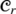of all “r-cornered” matrices as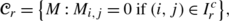and the set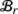of all “r-banded” matrices as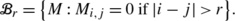Both sets are closed under matrix addition. It is also easy to check the following facts (for the admissible range of values for r and s):

1. (i)

If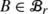and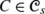, then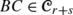and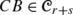.

2. (ii)

Ifand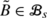, then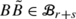.

3. (iii)

If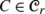and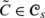, then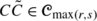.

4. (iv)

If, then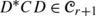.

Note that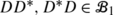and the commutator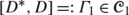. Define

$$\varGamma_r := \bigl(D^*D\bigr)^r - \bigl(DD^* \bigr)^r = \bigl(DD^*+\varGamma_1\bigr)^r - \bigl(DD^*\bigr)^r.$$

We expand out the first term (noting the non-commutativity), cancel (DD )r and see that every term that remains is a product of r terms (counting each DD as one term) each of which is either in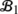or in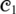. Repeated applications of (i), (ii), and (iii) yield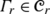.

We will now show by induction on r that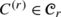for all r such that 2rm. The cases r=0 and r=1 hold trivially. Assume the statement holds for a given value of r. Since

$$C^{(r+1)} = D^* \bigl(C^{(r)} + \varGamma_r\bigr) D$$

and, property (iv) above now shows that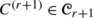. □

The next result, originally due to Weyl (see, e.g., [4, Theorem III.2.1]), will now allow us to estimate the eigenvalues of D r D r using the eigenvalues of (D D)r:

### Theorem A.2

(Weyl)

Let B and C be m×m Hermitian matrices where C has rank at most p and m>2p. Then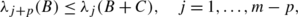(76)(77)

where we assume eigenvalues are in descending order.

We are now fully equipped to prove Proposition 3.2.

### Proof of Proposition 3.2

We set p=2r, B=(D D)r, and C=C (r)=D r D r−(D D)r in Weyl’s theorem. By Proposition A.1, C has rank at most 2r. Hence, we have the relations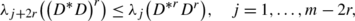(78)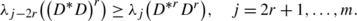(79)

Since λ j ((D D)r)=λ j (D D)r, this corresponds to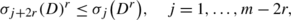(80)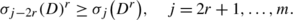(81)

For the remaining values of j, we will simply use the largest and smallest singular values of D r as upper and lower bounds. However, note that

$$\sigma_1\bigl(D^r\bigr) = \bigl\|D^r \bigr\|_{\mathrm{op}} \leq \|D\|_{\mathrm{op}}^{r} = \bigl( \sigma_1(D)\bigr)^r$$

and similarly

$$\sigma_m\bigl(D^r\bigr) = \bigl\|D^{-r} \bigr\|^{-1}_{\mathrm{op}} \geq \bigl\|D^{-1}\bigr\|_{\mathrm{op}}^{-r} = \bigl(\sigma_m(D)\bigr)^r.$$

Hence (80) and (81) can be rewritten together as

$$\sigma_{\min(j+2r,m)}(D)^r \leq\sigma_{j} \bigl(D^r\bigr) \leq \sigma_{\max(j-2r,1)}(D)^r,\quad j=1, \ldots,m.$$
(82)

Inverting these relations via (72), we obtain

$$\sigma_{\min(j+2r,m)}\bigl(D^{-1}\bigr)^r \leq \sigma_{j}\bigl(D^{-r}\bigr) \leq \sigma_{\max(j-2r,1)} \bigl(D^{-1}\bigr)^r,\quad j=1,\ldots,m.$$
(83)

Finally, to demonstrate the desired bounds of Proposition 3.2, we rewrite (74) via the inequality 2x/π≤sinxx for 0≤xπ/2 as

$$\frac{m+1/2}{\pi(j-1/2)} \leq\sigma_j\bigl(D^{-1} \bigr) \leq\frac{m+1/2}{2(j-1/2)},$$
(84)

and observe that min(j+2r,m)≍ r j and max(j−2r,1)≍ r j for j=1,…,m. □

### Remark

The constants c 1(r) and c 2(r) that one obtains from the above argument would be significantly exaggerated. This is primarily due to the fact that Proposition 3.2 is not stated in the tightest possible form. The advantage of this form is the simplicity of the subsequent analysis in Sect. 3.1. Our estimates of σ min(D r E) would become significantly more accurate if the asymptotic distribution of σ j (D r) is incorporated into our proofs in Sect. 3.1. However, the main disadvantage would be that the estimates would then hold only for all sufficiently large m.

## Rights and permissions

Reprints and Permissions

Güntürk, C.S., Lammers, M., Powell, A.M. et al. Sobolev Duals for Random Frames and ΣΔ Quantization of Compressed Sensing Measurements. Found Comput Math 13, 1–36 (2013). https://doi.org/10.1007/s10208-012-9140-x

• Revised:

• Accepted:

• Published:

• Issue Date:

### Keywords

• Quantization
• Finite frames
• Random frames
• Alternative duals
• Compressed sensing

• 41A46
• 94A12